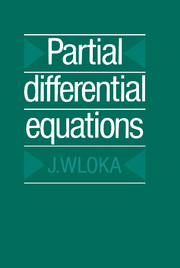Skip to content# Partial Differential Equations

## \$120.00 (X)

• Date Published: May 1987
• availability: Available
• format: Paperback
• isbn: 9780521277594

## \$120.00 (X) Paperback

### Request examination copy

Instructors may request a copy of this title for examination

Description
Product filter button
Description
Contents
Resources
Courses
• This book is a rigorous introduction to the abstract theory of partial differential equations. The main prerequisite is familiarity with basic functional analysis: more advanced topics such as Fredholm operators, the Schauder fixed point theorem and Bochner integrals are introduced when needed, and the book begins by introducing the necessary material from the theory of distributions and Sobolev spaces. Using such techniques, the author presents different methods available for solving elliptic, parabolic and hyperbolic equations. He also considers the difference process for the practical solution of a partial differential equation, emphasising that it is possible to solve them numerically by simple methods. Many examples and exercises are provided throughout, and care is taken to explain difficult points. Advanced undergraduates and graduate students will appreciate this self-contained and practical introduction.

## Customer reviews

### Not yet reviewed

Be the first to review

Review was not posted due to profanity

×

## , create a review

(If you're not , sign out)

Your review must be a minimum of 12 words.

×

## Product details

• Date Published: May 1987
• format: Paperback
• isbn: 9780521277594
• length: 532 pages
• dimensions: 227 x 151 x 34 mm
• weight: 0.774kg
• availability: Available

Preface
Part I. Sobolev Spaces:
1. Notation, basic properties, distributions
2. Geometric assumptions for the domain
3. Definitions and density properties for the Sobolev-Slobodeckii spaces
4. The transformation theorem and Sobolev spaces on differentiable manifolds
5. Definition of Sobolev spaces by the Fourier transformation and extension theorems
6. Continuous embeddings and Sobolev's lemma
7. Compact embeddings
8. The trace operator
9. Weak sequential compactness and approximation of derivatives by difference quotients
Part II. Elliptic Differential Operators:
10. Linear differential operators
11. The Lopatinskil-Sapiro condition and examples
12. Fredholm operators
13. The main theorem and some theorems on the index of elliptic boundary value problems
14. Green's formulae
15. The adjoint boundary value problem and the connection with the image space of the original operator
16. Examples
Part III. Strongly Elliptic Differential Operators and the Method of Variations:
17. Gelfand triples, the Law-Milgram, V-elliptic and V-coercive operators
18. Agmon's condition
19. Agmon's theorem: conditions for the V-coercion of strongly elliptic differential operators
20. Regularity of the solutions of strongly elliptic equations
21. The solution theorem for strongly elliptic equations and examples
22. The Schauder fixed point theorem and a non-linear problem
23. Elliptic boundary value problemss for unbounded regions
Part IV. Parabolic Differential Operators:
24. The Bochner integral
25. Distributions with values in a Hilbert space H and the space W
26. The existence and uniqueness of the solution of a parabolic differential equation
27. The regularity of solutions of the parabolic differential equation
28. Examples
Part V. Hyperbolic Differential Operators:
29. Existence and uniqueness of the solution
30. Regularity of the solutions of the hyperbolic differential equation
Part VI. Difference Processes for the Calculation of the Solution of the Partial Differential Equation:
32. Functional analytic concepts for difference processes
33. Difference processes for elliptic differential equations and for the wave equation
34. Evolution equations
References
Function and distribution spaces
Index.

• ## Author

J. Wloka

Not already registered? Create an account now. ×

### Sorry, this resource is locked

» Proceed

You are now leaving the Cambridge University Press website. Your eBook purchase and download will be completed by our partner www.ebooks.com. Please see the permission section of the www.ebooks.com catalogue page for details of the print & copy limits on our eBooks.

×